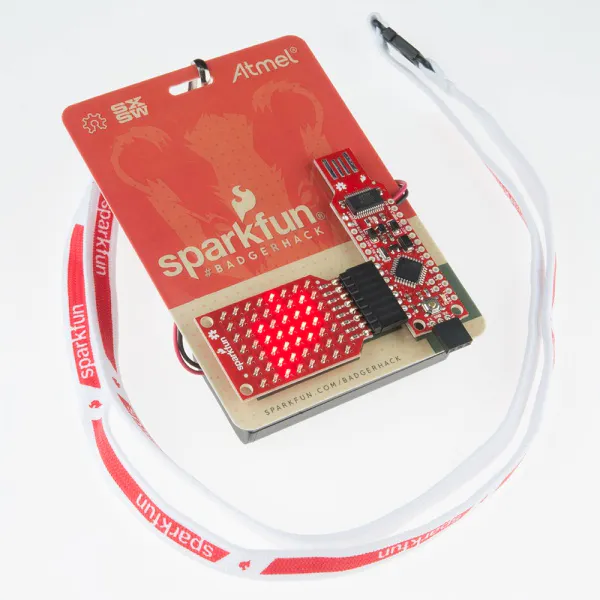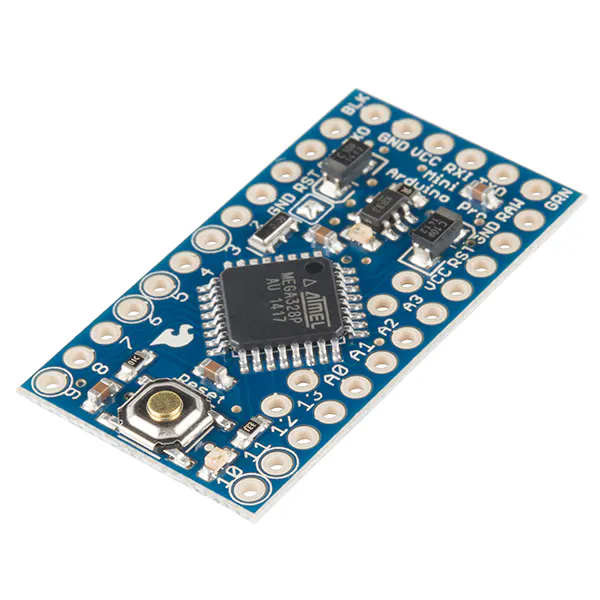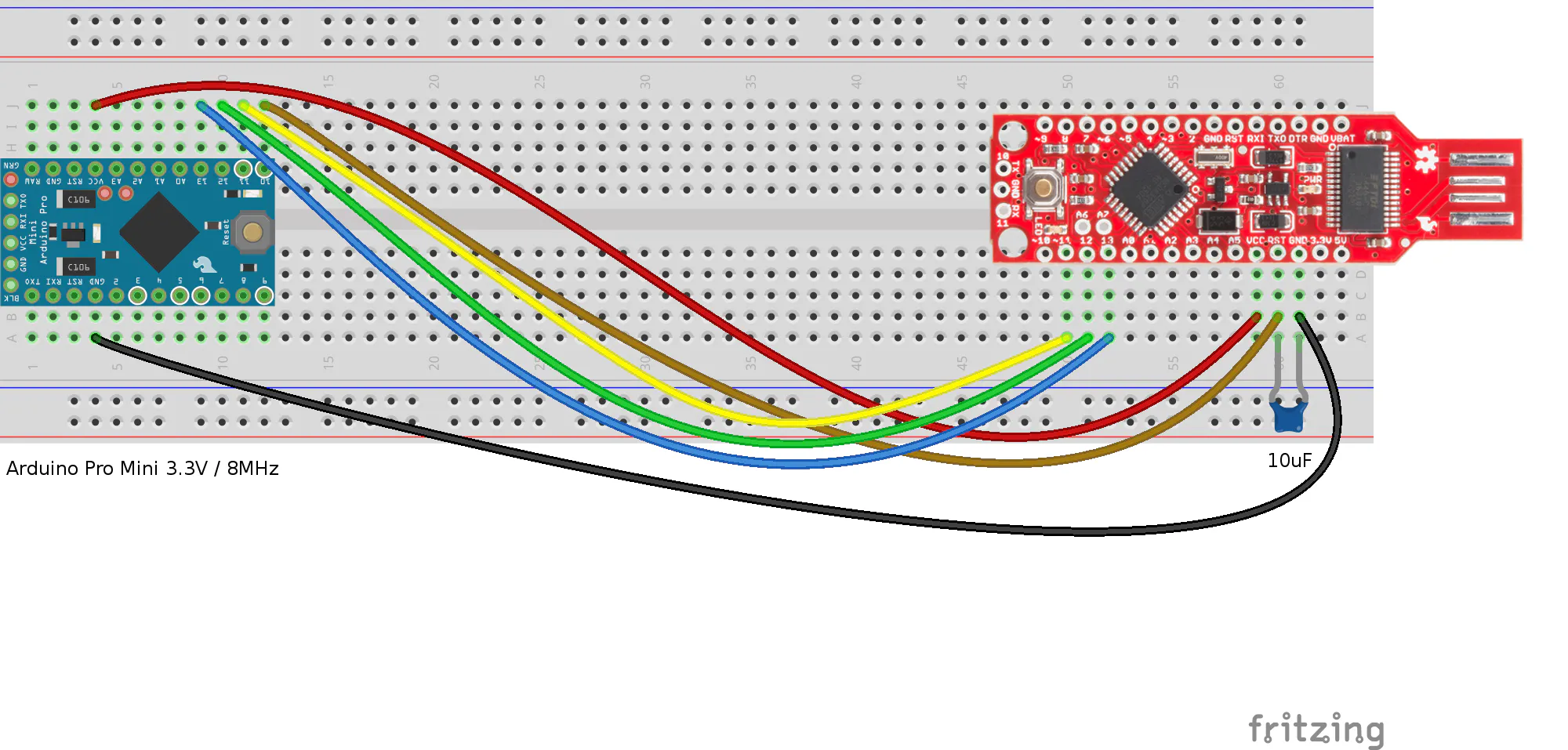How to upgrade the BadgerStick Arduino board by SparkFun, if you got one at SXSW 2015

IntermediateFull instructions provided1,713## Things used in this project

### Hardware components

×1SparkFun Arduino Pro Mini 328 - 3.3V/8MHz
×1

### Software apps and online services

 codebender

## Schematics

### Connecting the BadgerStick to an Arduino Pro Mini## Code

### Untitled file

Arduino
```// ArduinoISP version 04m3
// Copyright (c) 2008-2011 Randall Bohn
// If you require a license, see
//
// This sketch turns the Arduino into a AVRISP
// using the following arduino pins:
//
// pin name:    not-mega:         mega(1280 and 2560)
// slave reset: 10:               53
// MOSI:        11:               51
// MISO:        12:               50
// SCK:         13:               52
//
// Put an LED (with resistor) on the following pins:
// 9: Heartbeat   - shows the programmer is running
// 8: Error       - Lights up if something goes wrong (use red if that makes sense)
// 7: Programming - In communication with the slave
//
// 23 July 2011 Randall Bohn
// -Address Arduino issue 509 :: Portability of ArduinoISP
//
// October 2010 by Randall Bohn
// - Write to EEPROM > 256 bytes
// - Better use of LEDs:
// -- Flash LED_PMODE on each flash commit
// -- Flash LED_PMODE while writing EEPROM (both give visual feedback of writing progress)
// - Light LED_ERR whenever we hit a STK_NOSYNC. Turn it off when back in sync.
// - Use pins_arduino.h (should also work on Arduino Mega)
//
// October 2009 by David A. Mellis
//
// February 2009 by Randall Bohn
// - Added support for writing to EEPROM (what took so long?)
// Windows users should consider WinAVR's avrdude instead of the
// avrdude included with Arduino software.
//
// January 2008 by Randall Bohn
// - Thanks to Amplificar for helping me with the STK500 protocol
// - The AVRISP/STK500 (mk I) protocol is used in the arduino bootloader
// - The SPI functions herein were developed for the AVR910_ARD programmer

#include "pins_arduino.h"
#define RESET     SS

#define LED_HB    9
#define LED_ERR   8
#define LED_PMODE 7
#define PROG_FLICKER true

#define HWVER 2
#define SWMAJ 1
#define SWMIN 18

// STK Definitions
#define STK_OK      0x10
#define STK_FAILED  0x11
#define STK_UNKNOWN 0x12
#define STK_INSYNC  0x14
#define STK_NOSYNC  0x15
#define CRC_EOP     0x20 //ok it is a space...

void pulse(int pin, int times);

void setup() {
Serial.begin(19200);
pinMode(LED_PMODE, OUTPUT);
pulse(LED_PMODE, 2);
pinMode(LED_ERR, OUTPUT);
pulse(LED_ERR, 2);
pinMode(LED_HB, OUTPUT);
pulse(LED_HB, 2);
}

int error=0;
int pmode=0;
int here;
uint8_t buff; // global block storage

typedef struct param {
uint8_t devicecode;
uint8_t revision;
uint8_t progtype;
uint8_t parmode;
uint8_t polling;
uint8_t selftimed;
uint8_t lockbytes;
uint8_t fusebytes;
int flashpoll;
int eeprompoll;
int pagesize;
int eepromsize;
int flashsize;
}
parameter;

parameter param;

// this provides a heartbeat on pin 9, so you can tell the software is running.
uint8_t hbval=128;
int8_t hbdelta=8;
void heartbeat() {
if (hbval > 192) hbdelta = -hbdelta;
if (hbval < 32) hbdelta = -hbdelta;
hbval += hbdelta;
analogWrite(LED_HB, hbval);
delay(20);
}

void loop(void) {
// is pmode active?
if (pmode) digitalWrite(LED_PMODE, HIGH);
else digitalWrite(LED_PMODE, LOW);
// is there an error?
if (error) digitalWrite(LED_ERR, HIGH);
else digitalWrite(LED_ERR, LOW);

// light the heartbeat LED
heartbeat();
if (Serial.available()) {
avrisp();
}
}

uint8_t getch() {
while(!Serial.available());
}
void fill(int n) {
for (int x = 0; x < n; x++) {
buff[x] = getch();
}
}

#define PTIME 30
void pulse(int pin, int times) {
do {
digitalWrite(pin, HIGH);
delay(PTIME);
digitalWrite(pin, LOW);
delay(PTIME);
}
while (times--);
}

void prog_lamp(int state) {
if (PROG_FLICKER)
digitalWrite(LED_PMODE, state);
}

void spi_init() {
uint8_t x;
SPCR = 0x53;
x=SPSR;
x=SPDR;
}

void spi_wait() {
do {
}
while (!(SPSR & (1 << SPIF)));
}

uint8_t spi_send(uint8_t b) {
SPDR=b;
spi_wait();
}

uint8_t spi_transaction(uint8_t a, uint8_t b, uint8_t c, uint8_t d) {
uint8_t n;
spi_send(a);
n=spi_send(b);
//if (n != a) error = -1;
n=spi_send(c);
return spi_send(d);
}

if (CRC_EOP == getch()) {
Serial.print((char)STK_INSYNC);
Serial.print((char)STK_OK);
}
else {
error++;
Serial.print((char)STK_NOSYNC);
}
}

if (CRC_EOP == getch()) {
Serial.print((char)STK_INSYNC);
Serial.print((char)b);
Serial.print((char)STK_OK);
}
else {
error++;
Serial.print((char)STK_NOSYNC);
}
}

void get_version(uint8_t c) {
switch(c) {
case 0x80:
break;
case 0x81:
break;
case 0x82:
break;
case 0x93:
break;
default:
}
}

void set_parameters() {
// call this after reading paramter packet into buff[]
param.devicecode = buff;
param.revision   = buff;
param.progtype   = buff;
param.parmode    = buff;
param.polling    = buff;
param.selftimed  = buff;
param.lockbytes  = buff;
param.fusebytes  = buff;
param.flashpoll  = buff;
// ignore buff (= buff)
// following are 16 bits (big endian)
param.eeprompoll = beget16(&buff);
param.pagesize   = beget16(&buff);
param.eepromsize = beget16(&buff);

// 32 bits flashsize (big endian)
param.flashsize = buff * 0x01000000
+ buff * 0x00010000
+ buff * 0x00000100
+ buff;

}

void start_pmode() {
spi_init();
// following delays may not work on all targets...
pinMode(RESET, OUTPUT);
digitalWrite(RESET, HIGH);
pinMode(SCK, OUTPUT);
digitalWrite(SCK, LOW);
delay(50);
digitalWrite(RESET, LOW);
delay(50);
pinMode(MISO, INPUT);
pinMode(MOSI, OUTPUT);
spi_transaction(0xAC, 0x53, 0x00, 0x00);
pmode = 1;
}

void end_pmode() {
pinMode(MISO, INPUT);
pinMode(MOSI, INPUT);
pinMode(SCK, INPUT);
pinMode(RESET, INPUT);
pmode = 0;
}

void universal() {
int w;
uint8_t ch;

fill(4);
ch = spi_transaction(buff, buff, buff, buff);
}

void flash(uint8_t hilo, int addr, uint8_t data) {
spi_transaction(0x40+8*hilo,
data);
}
if (PROG_FLICKER) prog_lamp(LOW);
if (PROG_FLICKER) {
delay(PTIME);
prog_lamp(HIGH);
}
}

//#define _current_page(x) (here & 0xFFFFE0)
if (param.pagesize == 32)  return here & 0xFFFFFFF0;
if (param.pagesize == 64)  return here & 0xFFFFFFE0;
if (param.pagesize == 128) return here & 0xFFFFFFC0;
if (param.pagesize == 256) return here & 0xFFFFFF80;
return here;
}

void write_flash(int length) {
fill(length);
if (CRC_EOP == getch()) {
Serial.print((char) STK_INSYNC);
Serial.print((char) write_flash_pages(length));
}
else {
error++;
Serial.print((char) STK_NOSYNC);
}
}

uint8_t write_flash_pages(int length) {
int x = 0;
int page = current_page(here);
while (x < length) {
if (page != current_page(here)) {
commit(page);
page = current_page(here);
}
flash(LOW, here, buff[x++]);
flash(HIGH, here, buff[x++]);
here++;
}

commit(page);

return STK_OK;
}

#define EECHUNK (32)
uint8_t write_eeprom(int length) {
int start = here * 2;
int remaining = length;
if (length > param.eepromsize) {
error++;
return STK_FAILED;
}
while (remaining > EECHUNK) {
write_eeprom_chunk(start, EECHUNK);
start += EECHUNK;
remaining -= EECHUNK;
}
write_eeprom_chunk(start, remaining);
return STK_OK;
}
// write (length) bytes, (start) is a byte address
uint8_t write_eeprom_chunk(int start, int length) {
// this writes byte-by-byte,
// page writing may be faster (4 bytes at a time)
fill(length);
prog_lamp(LOW);
for (int x = 0; x < length; x++) {
delay(45);
}
prog_lamp(HIGH);
return STK_OK;
}

void program_page() {
char result = (char) STK_FAILED;
int length = 256 * getch();
length += getch();
char memtype = getch();
// flash memory @here, (length) bytes
if (memtype == 'F') {
write_flash(length);
return;
}
if (memtype == 'E') {
result = (char)write_eeprom(length);
if (CRC_EOP == getch()) {
Serial.print((char) STK_INSYNC);
Serial.print(result);
}
else {
error++;
Serial.print((char) STK_NOSYNC);
}
return;
}
Serial.print((char)STK_FAILED);
return;
}

return spi_transaction(0x20 + hilo * 8,
0);
}

for (int x = 0; x < length; x+=2) {
Serial.print((char) low);
Serial.print((char) high);
here++;
}
return STK_OK;
}

// here again we have a word address
int start = here * 2;
for (int x = 0; x < length; x++) {
int addr = start + x;
uint8_t ee = spi_transaction(0xA0, (addr >> 8) & 0xFF, addr & 0xFF, 0xFF);
Serial.print((char) ee);
}
return STK_OK;
}

char result = (char)STK_FAILED;
int length = 256 * getch();
length += getch();
char memtype = getch();
if (CRC_EOP != getch()) {
error++;
Serial.print((char) STK_NOSYNC);
return;
}
Serial.print((char) STK_INSYNC);
if (memtype == 'F') result = flash_read_page(length);
if (memtype == 'E') result = eeprom_read_page(length);
Serial.print(result);
return;
}

if (CRC_EOP != getch()) {
error++;
Serial.print((char) STK_NOSYNC);
return;
}
Serial.print((char) STK_INSYNC);
uint8_t high = spi_transaction(0x30, 0x00, 0x00, 0x00);
Serial.print((char) high);
uint8_t middle = spi_transaction(0x30, 0x00, 0x01, 0x00);
Serial.print((char) middle);
uint8_t low = spi_transaction(0x30, 0x00, 0x02, 0x00);
Serial.print((char) low);
Serial.print((char) STK_OK);
}
//////////////////////////////////////////
//////////////////////////////////////////

////////////////////////////////////
////////////////////////////////////
int avrisp() {
uint8_t data, low, high;
uint8_t ch = getch();
switch (ch) {
case '0': // signon
error = 0;
break;
case '1':
if (getch() == CRC_EOP) {
Serial.print((char) STK_INSYNC);
Serial.print("AVR ISP");
Serial.print((char) STK_OK);
}
break;
case 'A':
get_version(getch());
break;
case 'B':
fill(20);
set_parameters();
break;
case 'E': // extended parameters - ignore for now
fill(5);
break;

case 'P':
start_pmode();
break;
case 'U': // set address (word)
here = getch();
here += 256 * getch();
break;

case 0x60: //STK_PROG_FLASH
low = getch();
high = getch();
break;
case 0x61: //STK_PROG_DATA
data = getch();
break;

case 0x64: //STK_PROG_PAGE
program_page();
break;

break;

case 'V': //0x56
universal();
break;
case 'Q': //0x51
error=0;
end_pmode();
break;

break;

// expecting a command, not CRC_EOP
// this is how we can get back in sync
case CRC_EOP:
error++;
Serial.print((char) STK_NOSYNC);
break;

// anything else we will return STK_UNKNOWN
default:
error++;
if (CRC_EOP == getch())
Serial.print((char)STK_UNKNOWN);
else
Serial.print((char)STK_NOSYNC);
}
}
```

## Credits

### Georgitzikis Vasilis (tzikis)

2 projects • 1 follower